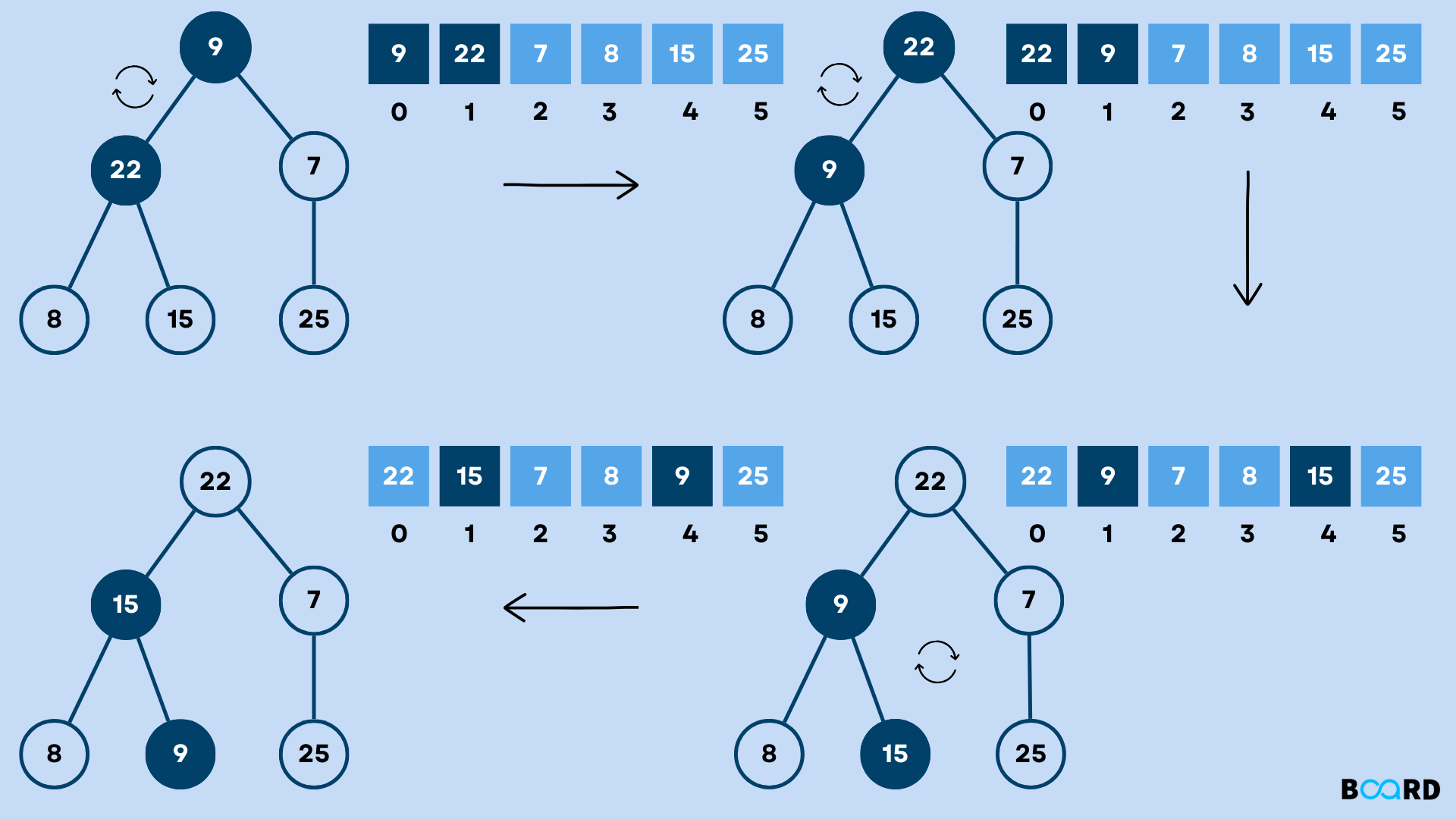# Heap Sort Algorithm## Introduction

Heap Sort is one of the most useful Sorting Algorithms which is based on comparison. It is based on the Heap Data Structure. This sorting technique first builds the max heap or min heap from the elements of the given array and stores the elements in the array in sorted order. Before knowing about the Heap Sort, let’s see what a heap is.

## What is Heap?

A Heap is a Complete Binary Tree in which the value at each node is either greater or smaller than its child node. There are two types of Heap:

• Max Heap: The value of each node is greater than its children.
• Min Heap: The value of each node is Smaller than its children.

It is clear that in the Max heap, the root node has the maximum value while in Min Heap, the root node has the Minimum value. Thus, for sorting an array, the minimum value is found and stored in the array then, heapify operation is performed again which gives the second minimum element. This process is repeated for the remaining elements.

## What is Heapify Operation?

Heapify is the operation of building a heap from the elements of an array. Heapify Operation can be used to build a max heap or min heap. The heapify operation starts from the non-leaf node whose index is (n/2)-1. The algorithm for the Heapify Operation is given below:

Heapify(arr, i):

1. L=leftchild(i)
2. R=rightchild(i)
3. If arr[L] > arr[i] and heapsize>1

largest=L

4. If arr[R]>arr[largest] and heapsize>1

largest=R

5. If (i) is not the largest:

swap(arr[i], largest)

## Heap Sort Algorithm

The basic idea of this sorting technique is given below:

1. First, convert the array into a heap using the heapify algorithm.
2. Swap the root element with the element at the last position of the heap.
3. Reduce the heap size by 1
4. Heapify the new root element again and repeat the process till the size of the heap is greater than 1.

Let’s convert the above algorithm into a Heap Sort Program in C. One of the most common confusion regarding heap sort is that it uses a binary tree or heap to sort the list. But, this is not true. It doesn't use any other data structure except the input array. That’s why It is an in-place sorting algorithm which means that it uses constant space for producing the output. This will be more clear as we go ahead with the algorithm.

There will be two functions, the first function will heapify the array and another function will sort the array. For convenience, we are creating another function ‘swap’ which will swap the elements as there is no built-in function in C to swap the elements.

Suppose the array is [1,12,9,5,6,10], the Heapify Process converts it into [12,6,10,5,1,9] which is the max heap (maximum element at the top). Then, 12 is swapped with 9. Therefore, [12,6,10,5,1,9] becomes [9,6,10,5,1,12].

After this, the heap size is reduced by 1 and the new root (9) is passed to the heapify function to get the maximum element on top. Thus, the array becomes [10,6,9,5,1,12] with 10 (maximum at the top). Then 10 is swapped with 1 to form [1,6,9,5,10,12]. This process is repeated till the heap size is greater than one. As a result, the array is sorted.

## Code

Below is the C code for the Heap Sort Algorithm:

 #include void heapify(int arr[], int size, int current); void heapSort(int arr[], int size); void swap(int* a, int* b); int main(){    int arr[]= {12,6,10,5,1,9};    int size= sizeof(arr)/sizeof(arr);    heapSort(arr, size);    printf("Sorted array is:\n");    for (int i = 0; i < size; i++)    {        printf("%d\t", arr[i]);    } }//heapify function to build max heap from the array elementsvoid heapify(int arr[], int size, int current){    // let's assume that current root is largest    int largest = current;    // initialize left and right child of the root element (left and right denotes the position of children)    int left= 2*current+1;      int right= 2*current+2;    //find the largest among root, left and right child    if(leftarr[largest]){        largest=left;    }    if(rightarr[largest]){        largest=right;    }    // swap the root(current element) with the largest root    if(current!=largest ){        swap(&arr[current], &arr[largest]);        //heapify the new root again to get maximum on the top position        heapify(arr, size, largest);    }}// heap sort function to sort the elementsvoid heapSort(int arr[], int size){    //build the max heap using heapify    //always start from ((size/2)-1)th node    for(int i= (size/2)-1;i>=0;i--){        heapify(arr, size, i);    }    //extract elements from heap and store at last position of heap i.e. arr    for(int i=size-1;i>=0;i--){        swap(&arr, &arr[i]);        //heapify the root element again to get maximum element on top        heapify(arr, i, 0 );    }}//swap function to interchange the elementsvoid swap(int* a, int* b){    int t= *a;    *a=*b;    *b=t;}

Output

 Sorted array is:1 5 6 9 10 12
write your code here: Coding Playground